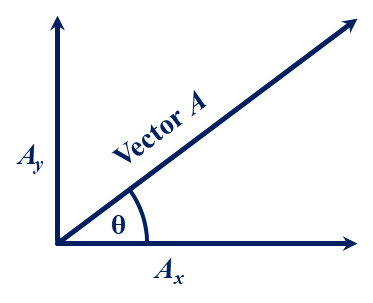﻿ Week 5 Doc O's FHS Physics Assignment Page

Week 5:  3 October to 7 October 2022

30 September 2023:

In Class:  Newton's First Law:  The Law of Inertia.

Expectations:  Students will be able to predict the motion of objects and identify which object have greater inertia.

Due:  Pages 1:148-149 ( 102 – 105 ).

Homework:  Pages 1:149-150 (106 – 112).

3 October 2022:

In Class:  Newton's Second Law:

• a =
•  Fnet
m
• a = acceleration
Fnet = net force
m = mass
• Expectations:  Students will solve problems using the Newton's 2nd Law equation.

Lab 2a:
Finish the Orienteering Lab (graphical vectors) Part b.

Expectations:  Students will solve for the resultant of vectors graphically.

Due:  Pages 1:149-150 (106 – 112).

Homework:  Pages 1:150-152 (113 – 125).

4 October 2022:

In Class:  Newton's Third Law:  Action – Reaction Law.

Expectations:  Students will solve problems using the Newton's 3rd Law equation.

Due:  Pages 1:150-152 (113 – 125).

Homework:  Pages 1:152-153 (126 – 134).

5 October 2022:

In Class:  Motion in two dimensions.

Expectations:  Students will be able to solve problems with motion in two dimensions using vertical components.

Due:  Pages 1:152-153 (126 – 134).

Homework:  Pages 1:153-156 (135 – 153).

6 October 2022:

No Class:  Physics does not meet on Thursday.

7 October 2022:

In Class:  Projectiles Fired at an Angle.

• Ay = A sin θ
Ax = A cos θ
• A = Any vector
Ay = Vertical component of A
Ax = Horizontal component of A
θ = Angle from A to horizontal
•• Expectations:  Students will resolve a vector into its vertical and horizontal components and solve problems to find range, altitude, and time in the air for projectiles fired at different angles to the ground.

Due:  Pages 1:153-156 (135 – 153).

Homework:  Pages 1:155-156 (154 – 165).

Note:  1st quarter ends 9 November 2023.